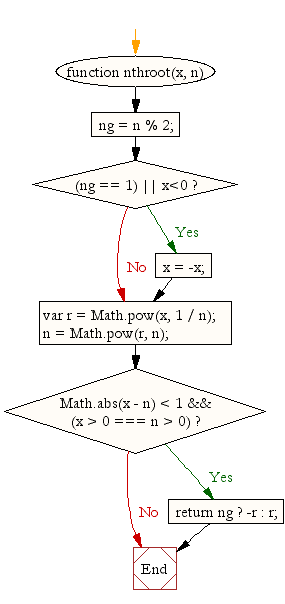# JavaScript: Calculate the nth root of a number

## JavaScript Math: Exercise-26 with Solution

Write a JavaScript function to calculate the nth root of a number.

Test Data :
console.log(nthroot(64, 2));
8
console.log(nthroot(64, -2));
0.125

Sample Solution:-

HTML Code:

``````<!DOCTYPE html>
<html>
<meta charset="utf-8">
<title>JavaScript function to calculate the nth root of a number</title>
<body>

</body>
</html>
```
```

JavaScript Code:

``````function nthroot(x, n)
{
ng = n % 2;
if((ng == 1) || x<0)
x = -x;
var r = Math.pow(x, 1 / n);
n = Math.pow(r, n);

if(Math.abs(x - n) < 1 && (x > 0 === n > 0))
return ng ? -r : r;
}
console.log(nthroot(64, 2));
console.log(nthroot(64, -2));
```
```

Sample Output:

```8
0.125
```

Pictorial Presentation:Flowchart:Live Demo:

See the Pen javascript-math-exercise-26 by w3resource (@w3resource) on CodePen.

Improve this sample solution and post your code through Disqus

What is the difficulty level of this exercise?

Test your Programming skills with w3resource's quiz.

﻿